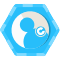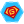## 45个实用的JavaScript黑科技 原

众所周知，Javascript是全球最流行的语言之一，它涉足Web开发，移动端开发（PhoneGap 、 Appcelerator ），服务端开发（ Nodejs 、 Wakanda ），还有多种第三方实现（CoffeeScript这种）。此外Javascript还是许多开发者进入编程世界所接触的第一门语言。它既可以在浏览器中简单的弹出一个alert窗口，也能达到控制机器人这种复杂的程度（比如 nodebot 、nodruino）。现在那些能够熟练编写结构清晰、性能卓越的Javascript开发者们，已经成为招聘市场上炙手可热的应聘者了。

在这篇文章中，我将向你展示一系列Javascript相关的小技巧和一些最佳实践。除了少数几个示例，大部分示例都可以在浏览器环境或者服务端环境适用。

## 2、使用 `===` 来代替 `==` 进行判等

`==` 和 `!=` 操作符会在某些情况下自动进行类型转化。但是 `===` 和 `!==` 不会做自动转化，它们在做比较时，会同时比较数据类型和值，这也使得 `===` 和 `!==` 要比`==` 和 `!=` 的速度要快。

``` === 10    // is false
  == 10    // is true
'10' == 10     // is true
'10' === 10    // is false
[] == 0        // is true
[] ===  0      // is false
'' == false    // is true but true == "a" is false
'' ===   false // is false```

## 5、使用对象构造器

```functionPerson(firstName, lastName){
this.firstName =  firstName;
this.lastName = lastName;
}

## 6、使用 `typeof` 、 `instanceof` 、 `constructor` 时要谨慎小心

• `typeof` ：JavaScript的一元操作符，用于以字符串的形式返回变量的原始类型，注意， `typeof null` 也会返回 `object` ，大多数的对象类型（数组 `Array` 、时间 `Date` 等）也会返回 `object`
• `constructor` ：对象（函数）的内部原型属性，它是可写的（可以被重写）
• `instanceof` ：JavaScript操作符，会在原型链中的构造器中搜索，找到则返回`true` ，否则返回 `false` （常用于判断某一个对象是否是某个构造器或者其父类构造器的实例）
```var arr = ["a", "b", "c"];
typeof arr;   // return "object"
arr instanceof Array // true
arr.constructor();  // []```

## 7、学会使用自调用函数

```(function(){
// some private code that will be executed automatically
})();

(function(a,b){
var result = a+b;
return result;
})(10,20);```

## 8、随机从数组中取出一个元素

```var items = [12, 548 , 'a' , 2 , 5478 , 'foo' , 8852, , 'Doe' , 2145 , 119];
var randomItem = items[Math.floor(Math.random() * items.length)];```

## 9、从一个指定的范围中取出一个随机数

`var x = Math.floor(Math.random() * (max - min + 1)) + min;`

## 10、生成一个从0开始到指定数字的序列

```var numbersArray = [] , max = 100;
for( var i=1; numbersArray.push(i++) < max;);  // numbers = [1,2,3 ... 100]```

## 11、生成一个随机的字母数字序列

```functiongenerateRandomAlphaNum(len) {
var rdmString = "";
for( ;rdmString.length < len; rdmString  += Math.random().toString(36).substr(2));
return  rdmString.substr(0, len);
}```

`toString()` 方法可以接受一个参数表示数字进制。而36进制刚好可以使用a-z和0-9这些字符。所以此方法可以用于生成简单的随机串。

## 12、打乱一个数字数组的顺序

```var numbers = [5, 458 , 120 , -215 , 228 , 400 , 122205, -85411];
numbers = numbers.sort(function(){ return Math.random() - 0.5});
/* the array numbers will be equal for example to [120, 5, 228, -215, 400, 458, -85411, 122205]  */```

## 13、字符串去空格

```String.prototype.trim = function() {
returnthis.replace(/^\s+|\s+\$/g, "");
};```

## 14、将一个数组追加到另一个数组中

```var array1 = [12 , "foo" , {name "Joe"} , -2458];
var array2 = ["Doe" , 555 , 100];
Array.prototype.push.apply(array1, array2);
/* array1 值为  [12 , "foo" , {name "Joe"} , -2458 , "Doe" , 555 , 100] */```

## 15、将 `argruments` 转换成数组

`var argArray = Array.prototype.slice.call(arguments);`

## 16、检验一个参数是否为数字

```functionisNumber(n){
return !isNaN(parseFloat(n)) && isFinite(n);
}```

## 17、检验一个参数是否为数组

```functionisArray(obj){
return Object.prototype.toString.call(obj) === '[object Array]' ;
}```

`Array.isArray(obj); // its a new Array method`

```var myFrame = document.createElement('iframe');
document.body.appendChild(myFrame);

var myArray = window.frames[window.frames.length-1].Array;
var arr = new myArray(a,b,10); // [a,b,10]// myArray 的构造器已经丢失，instanceof 的结果将不正常// 不同iframe中的构造器是不能共享的
arr instanceofArray; // false```

## 18、取出一个数组中的最大值和最小值

```var numbers = [5, 458 , 120 , -215 , 228 , 400 , 122205, -85411];
var maxInNumbers = Math.max.apply(Math, numbers);
var minInNumbers = Math.min.apply(Math, numbers);```

## 19、清空一个数组

```var myArray = [12 , 222 , 1000 ];
myArray.length = 0; // myArray will be equal to [].```

## 20、不用使用 `delete` 关键字来移除一个数组元素

```var items = [12, 548 ,'a' , 2 , 5478 , 'foo' , 8852, , 'Doe' ,2154 , 119 ];
items.length; //return11delete items; //return true
items.length; //return11
/* items will be equal to [12, 548, "a", undefined × 1, 5478, "foo", 8852, undefined × 1, "Doe", 2154,       119]   */```

```var items = [12, 548 ,'a' , 2 , 5478 , 'foo' , 8852, , 'Doe' ,2154 , 119 ];
items.length; //return11
items.splice(3,1) ;
items.length; //return10
/* items 结果为 [12, 548, "a", 5478, "foo", 8852, undefined × 1, "Doe", 2154, 119] */```

## 21、可以通过操作数组长度 `length` 来截断一个数组

```var myArray = [12 , 222 , 1000 , 124 , 98 , 10 ];
myArray.length = 4; // myArray will be equal to [12 , 222 , 1000 , 124].```

```myArray.length = 10; // the new array length is 10
myArray[myArray.length - 1] ; // undefined```

## 22、在条件中使用 `&&` 及 `||` 进行短语判断

```var foo = 10;
foo == 10 && doSomething(); // is the same thing asif (foo == 10) doSomething();
foo == 5 || doSomething(); // is the same thing asif (foo != 5) doSomething();```

`||` 还用于给函数参数设置默认值，比如

```functiondoSomething(arg1){
arg1 = arg1 || 10; // arg1 will have 10 as a default value if it’s not already set
}```

## 23、使用 `map()` 对数组进行遍历操作

```var squares = [1,2,3,4].map(function (val) {
return val * val;
});
// squares will be equal to [1, 4, 9, 16]```

## 24、保留指定位数的小数点

```var num = 2.443242342;
num = num.toFixed(4);  // num will be equal to 2.4432```

## 25、浮点数计算问题

`0.1 + 0.2 === 0.3// is false 9007199254740992 + 1// is equal to 9007199254740992  9007199254740992 + 2// is equal to 9007199254740994`

## 26、使用 `for...in` 来遍历对象的属性

```for (var name inobject) {
if (object.hasOwnProperty(name)) {
// do something with name
}
}```

## 27、逗号运算符

```var a = 0;
var b = ( a++, 99 );
console.log(a);  // a will be equal to 1
console.log(b);  // b is equal to 99```

## 28、缓存临时变量用于避免再次计算或者查询

```var navright = document.querySelector('#right');
var navleft = document.querySelector('#left');
var navup = document.querySelector('#up');
var navdown = document.querySelector('#down');```

## 29、提前检查传入 `isFinite()` 的参数

```isFinite(0/0) ; //false
isFinite("foo"); //false
isFinite("10"); //true
isFinite(10);   //true
isFinite(undefined);  //false
isFinite();   //false
isFinite(null);  //true  注意这里！！！```

## 30、避免对数组进行负值索引

```var numbersArray = [1,2,3,4,5];
varfrom = numbersArray.indexOf("foo") ;  // from is equal to -1
numbersArray.splice(from,2);    // will return ```

## 31、使用 `JSON` 来进行序列化和反序列化

```var person = {name :'Saad', age : 26, department : {ID : 15, name : "R&D"} };
var stringFromPerson = JSON.stringify(person);
/* stringFromPerson is equal to "{"name":"Saad","age":26,"department":{"ID":15,"name":"R&D"}}"   */var personFromString = JSON.parse(stringFromPerson);
/* personFromString is equal to person object  */```

## 32、避免使用 `eval()` 和函数构造器

`eval()` 和函数构造器（ `Function` consturctor）的开销都比较大，每次调用JavaScript引擎都要将源代码转换为可执行的代码。

```var func1 = new Function(functionCode);
var func2 = eval(functionCode);```

## 34、避免使用 `for...in` 遍历数组

```var sum = 0;
for (var i in arrayNumbers) {
sum += arrayNumbers[i];
}```

```var sum = 0;
for (var i = 0, len = arrayNumbers.length; i < len; i++) {
sum += arrayNumbers[i];
}```

`for (var i = 0; i < arrayNumbers.length; i++)`

## 35、给 `setTimeout()` 及 `setInterval()` 传递函数而不是字符串更好

```setInterval('doSomethingPeriodically()', 1000);
setTimeout('doSomethingAfterFiveSeconds()', 5000);```

```setInterval(doSomethingPeriodically, 1000);
setTimeout(doSomethingAfterFiveSeconds, 5000);```

## 37、在 `switch/case` 中使用数字范围进行分界

```functiongetCategory(age) {
var category = "";
switch (true) {
case isNaN(age):
category = "not an age";
break;
case (age >= 50):
category = "Old";
break;
case (age <= 20):
category = "Baby";
break;
default:
category = "Young";
break;
};
return category;
}
getCategory(5);  // will return "Baby"```

## 38、为创建的对象指定原型

```functionclone(object) {
functionOneShotConstructor(){};
OneShotConstructor.prototype = object;
returnnew OneShotConstructor();
}
clone(Array).prototype ;  // []```

## 39、html转义函数

```functionescapeHTML(text) {
var replacements= {'<': '<', '>': '>', '&': '&', '"': '"'};
return text.replace(/[<>&"]/g, function(character) {
return replacements[character];
});
}```

## 40、不要在循环中使用 `try...catch...finally`

`try...catch...finally` 在捕获一个异常时，会创建一个运行时环境的子作用域。而异常变量的生命周期仅限在这个运行时的子作用域。

```varobject = ['foo', 'bar'], i;
for (i = 0, len = object.length; i <len; i++) {
try {
// do something that throws an exception
} catch (e) {
// handle exception
}
}```

```varobject = ['foo', 'bar'], i;
try {
for (i = 0, len = object.length; i <len; i++) {
// do something that throws an exception
}
} catch (e) {
// handle exception
}```

## 41、使用 `XMLHttpRequests` 时注意设置超时参数

```var xhr = new XMLHttpRequest ();
clearTimeout(timeout);
// do something with response data
}
}
var timeout = setTimeout( function () {
xhr.abort(); // call error callback
}, 60*1000/* timeout after a minute */ );
xhr.open('GET', url, true);
xhr.send();```

## 42、处理websocket超时

```var timerID = 0;

functionkeepAlive() {
var timeout = 15000;
webSocket.send('');
}
timerId = setTimeout(keepAlive, timeout);
}

functioncancelKeepAlive() {
if (timerId) {
cancelTimeout(timerId);
}
}```

`keepAlive()` 方法应该被添加在 `webSOcket` 连接的 `onOpen()` 方法的最后，而`cancelKeepAlive()` 添加在 `onClose()` 方法的最后。

## 43、记住：原生操作肯定比函数调用要效率高

```var min = Math.min(a,b);
A.push(v);```

`var min = a < b ? a:b; A[A.length] = v;`

## 45、与其他JavaScript类库冲突的解决方案

`\$(document).ready(function() {`

`   var` `\$jq = jQuery.noConflict();`

`   \$jq('#id').show();`

`});`### 孟飞阳### 评论(0)

2018/05/30
0
0

Fundebug
2019/07/26
0
0

1、单行写一个评级组件 "★★★★★☆☆☆☆☆".slice(5 - rate, 10 - rate);定义一个变量rate是1到5的值，然后执行上面代码，看图 才发现插件什么的都弱爆了 来源：来自知乎用户蜗牛老湿的回...

osc_jww09jef
2018/06/27
1
0

2018/09/03
0
0

VUE element 饿了吗vue2.0组件 http://element.eleme.io/#/zh-CN/resource vue开发步骤： 1 http://www.jianshu.com/p/5ba253651c3b 2 1）http://www.jianshu.com/p/5ba253651c3b 2）http://......

mircle
2017/05/27
11
0

PHP实战之文件上传与下载

27分钟前
30
0
rebar3 的使用

SummerGao
29分钟前
20
0

go4it
31分钟前
18
0

fyin1314
33分钟前
23
0

fairy1674
38分钟前
22
0